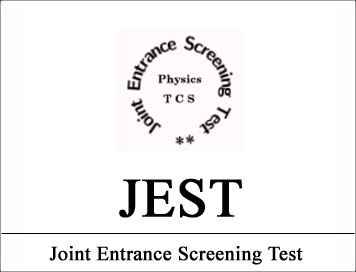# (Syllabus) Joint Entrance Screening Test (JEST)## (Syllabus) Joint Entrance Screening Test (JEST)

Mathematical methods

Vector algebra and vector calculus, tensors, curvilinear coordinate systems, linear algebra; Linear differential equations, elements of Sturm–Liouville theory; Special functions; Complex analysis; Fourier series and Fourier transforms, Laplace transforms; Elementary properties of discrete groups; Elements of probability theory, error analysis.

Classical mechanics

Newton’s laws, conservation of energy and momentum, collisions; generalized coordinates, principle of least action, Lagrangian and Hamiltonian formulations of mechanics; symmetry and conservation laws; central force problem, Kepler problem; small oscillations and normal modes; special relativity in classical mechanics.

Electromagnetism & optics

Electrostatics and magnetostatics, boundary value problems, multipole expansion; fields in conducting, dielectric, diamagnetic and paramagnetic media; Faraday’s law and time varying fields; displacement current; Maxwell’s equations; energy and momentum of electromagnetic fields; propagation of plane electromagnetic waves, reflection, refraction; electromagnetic waves in dispersive and conducting media; diffraction, interference, polarization.

Quantum mechanics

Uncertainty principle; Schrodinger equation; central potentials, hydrogen atom; orbital and spin angular momenta, addition of angular momenta; matrix formulation of quantum theory, unitary transformations, Hermitian operators; variational principle, time independent perturbation theory, time dependent perturbation theory.

Thermodynamics & statistical physics

Laws of thermodynamics, work and heat, thermodynamic potentials; Elements of kinetic theory; Maxwell’s relations; statistical ensembles; partition function; classical ideal gas, harmonic oscillators; classical and quantum statistics; Fermi and Bose gases; black body radiation; statistics of paramagnetism

Electronics

Basics of semiconductor; p-n junctions, diodes, transistors; LCR circuits, rectifiers, amplifiers, active filters and oscillators; basics of OPAMPs and their applications; basics of digital electronics.